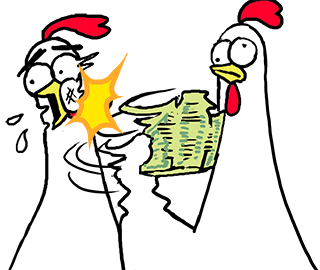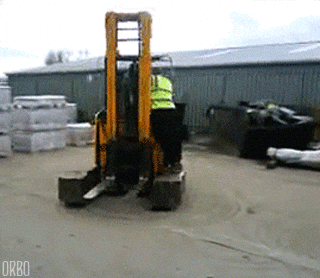## DEV Community is a community of 722,735 amazing developers

We're a place where coders share, stay up-to-date and grow their careers.ronsoak

Posted on

# The R.A.G (Redshift Analyst Guide): Understanding the Query Plan (Explain)

Welcome to the R.A.G, a guide about Amazon's Redshift Database written for the Analyst's out there in the world who use it.

### Previously on the R.A.G....

Redshift has the ability to explain to you how it's going to interpret the query you are about to run, going so far as to estimate how hard it's going to be, how much data it's going to crunch, and what moving around of data it's going to have to do.

This explanation (Query Plan) can help you understand the cost your query is going to have on Redshift which may help give you some tips on how to improve it.Example Query plan from AWS

``````QUERY PLAN
XN Merge  (cost=1015345167117.54..1015345167544.46 rows=1000 width=103)
Merge Key: category.catname, sum(sales.pricepaid)
->  XN Network  (cost=1015345167117.54..1015345167544.46 rows=170771 width=103)
->  XN Sort  (cost=1015345167117.54..1015345167544.46 rows=170771 width=103)
Sort Key: category.catname, sum(sales.pricepaid)
->  XN HashAggregate  (cost=15345150568.37..15345152276.08 rows=170771 width=103)
Filter: (sum(pricepaid) > 9999.00)
->  XN Hash Join DS_BCAST_INNER  (cost=742.08..15345146299.10 rows=170771 width=103)
Hash Cond: ("outer".catid = "inner".catid)
->  XN Hash Join DS_BCAST_INNER  (cost=741.94..15342942456.61 rows=170771 width=97)
Hash Cond: ("outer".dateid = "inner".dateid)
->  XN Hash Join DS_BCAST_INNER  (cost=737.38..15269938609.81 rows=170766 width=90)
->  XN Hash Join DS_BCAST_INNER  (cost=112.50..3272334142.59 rows=170771 width=84)
Hash Cond: ("outer".venueid = "inner".venueid)
->  XN Hash Join DS_BCAST_INNER  (cost=109.98..3167290276.71 rows=172456 width=47)
Hash Cond: ("outer".eventid = "inner".eventid)
->  XN Merge Join DS_DIST_NONE  (cost=0.00..6286.47 rows=172456 width=30)
Merge Cond: ("outer".listid = "inner".listid)
->  XN Seq Scan on listing  (cost=0.00..1924.97 rows=192497 width=14)
->  XN Seq Scan on sales  (cost=0.00..1724.56 rows=172456 width=24)
->  XN Hash  (cost=87.98..87.98 rows=8798 width=25)
->  XN Seq Scan on event  (cost=0.00..87.98 rows=8798 width=25)
->  XN Hash  (cost=2.02..2.02 rows=202 width=41)
->  XN Seq Scan on venue  (cost=0.00..2.02 rows=202 width=41)
->  XN Hash  (cost=499.90..499.90 rows=49990 width=14)
->  XN Seq Scan on users  (cost=0.00..499.90 rows=49990 width=14)
->  XN Hash  (cost=3.65..3.65 rows=365 width=11)
->  XN Seq Scan on date  (cost=0.00..3.65 rows=365 width=11)
->  XN Hash  (cost=0.11..0.11 rows=11 width=10)
->  XN Seq Scan on category  (cost=0.00..0.11 rows=11 width=10)
``````

## CostAs you can see, the word cost is found everywhere in the explain plan. Cost isn't the exact cost of how long it will run end to end, but is instead the relative cost of what it will take to execute the parts of the query.

So what does '1015345167117.54..1015345167544.46 rows=1000 width=103' actually tell you?

Well let's start with the REALLY long number, for one it's actually two numbers separated by two decimal points. So in this example it's 1015345167117.54 & 1015345167544.46 . The first number is the cost to get just the FIRST row of the operation, with the second being the cost to complete the whole job. The costs are cumulative as you go from bottom to top. So the top value is the total cost that includes all the ones below.

Lets break down some theoretical cost scenarios:

• First cost is low, second is high: This means that it's easy to get to the data but hard to complete the whole thing, this may just indicate a huge data set that it just needs to slug through. Not necessarily a bad thing, think of using some basic logic against a very large table . It could be an indication that some of your logic has an unreasonably high cost for what your wanting it to do, maybe you've saved a date field in a VARCHAR and Redshift is doing unnecessary conversion in the background.
• First and second cost are low: This is a good thing, don't change a thing.
• First and second cost are high (but both different): This indicates possibly a bad join, where two large tables are being joined on each other and/or heavy amounts of logic are being applied to both tables. Or possibly you are including far too many actions in a single query, remember to keep code simple.
• First cost is high, second is about equal.: This possibly indicates an overly complex query where it takes a lot of processing just to get the first row but once it has that it's not exponentially longer to complete the task. Think of searching a very large table for specifically three things without adding any other criteria.

Second is the rows part of the cost. This is an estimated amount of rows to return and relies heavily on table metadata, so tables that haven't been analysed in a while will return false values to the query plan and can lead to a bad query plan being executed. Row count is the closest thing to understanding how to improve the query as you can possibly add additional logic to bring that row count down.

Last is the width, this refers to the size cost of the columns being returned in bytes which is why it's recommend that you only bring back the rows you need. The only way to reduce this is to select fewer columns.

## Sequential Scan

Where you see this, this means that Redshift will scan the entire object (table, cte, sub-query) all rows and all columns checking for the criteria you have specified. This is why it's important to only be dealing with tables that are as small in both rows and columns as possible to speed up query time. In scenarios where you are hitting a source table with a sequential scan, which is unavoidable as you will always need to go a source table at least once, this is where you need to be taking advantage of the table's dist key and sort key as those are the only ways to hit the table as fast as possible.

## Inner and Outer

The EXPLAIN output also references inner and outer tables. The inner table is scanned first, and appears nearer the bottom of the query plan. The inner table is the table that is probed for matches. It is usually held in memory, is usually the source table for hashing, and if possible, is the smaller table of the two being joined. The outer table is the source of rows to match against the inner table. It is usually read from disk. The query optimizer chooses the inner and outer table based on database statistics from the latest run of the ANALYZE command. The order of tables in the FROM clause of a query doesn't determine which table is inner and which is outer.

## Join Types### Merge Join

In a merge join both tables are perfect for each other. Which means that the join condition on each side is the dist key and the sort key. Meaning both tables perfectly line up without any meddling needed.

This is the best join. Though rare to pull off.

### Hash Join

In a hash join, the join conditions aren't perfect for each other but Redshift can mange with a bit of work. So what Redshift does is look at both tables and between them creates a hash table which is like a lookup table that sits in the middle.

Once Redshift has created the hash table it can then do its job and match the two.

Obviously a Merge Join is better, but a Hash Join is fine if you can't swing a Merge, and is very favorable over a Nested Loop.

### Nested Loop Join

A nested loop occurs when a hash table can't be created between the two. This occurs when you use conditional criteria in the join, like between or greater than.

This will require the database to check every value in the left table against every value in the right table. The complexity of a Nested Loop Join would be “quadratic”, in that you need to do about N*N (or N²) different operations to process the join. Not great! Nested Loop Joins don’t hold up when you’re joining million-row tables together – your database might end up needing to complete trillions of operations to execute that joinWhen Redshift has to do a join, it may have to move the data around its nodes to complete the join being asked of it. The task of moving that data around can take up time and so obviously if you can avoid this then you can speed up your query.

In a broadcast, the data values from one side of a join are copied from each compute node to every other compute node, so that every compute node ends up with a complete copy of the data.

If this is occurring you will see the phrase DS_BCAST_INNER in the explain plan.

### Redistribution

In a redistribution, participating data values are sent from their current slice to a new slice (possibly on a different node). Data is typically redistributed to match the distribution key of the other table participating in the join if that distribution key is one of the joining columns.

If redistribution is occurring, you will see the following phrases:

• DS_DIST_ALL_NONE: No redistribution is required, because the inner table has already been distributed to every node using DISTSTYLE ALL.
• DS_DIST_NONE: No tables are redistributed. Collocated joins are possible because corresponding slices are joined without moving data between nodes.
• DS_DIST_INNER: The inner table is redistributed.
• DS_DIST_OUTER: The outer table is redistributed.
• DS_DIST_ALL_INNER: The entire inner table is redistributed to a single slice because the outer table uses DISTSTYLE ALL.
• DS_DIST_BOTH: Both tables are redistributed.

## Discussion (2)"The inner table is scanned first, and appears nearer the bottom of the query plan." Gold information, thanks. The only place I could find in the docs that vaguely hints that the lower-down query is the inner is here docs.amazonaws.cn/en_us/redshift/l...

In terms of the join operations, nested-loop, hash, merge, it's not so much that nested-loop is always bad. Sure the algorithm is quadratic, but the complexity of finding the optimal query plan is (probably) NP hard. There's a threshold where creating a plan can take too long, perhaps longer than just running a sub-optimal plan. If the estimated rowcount ( statistics are king! run vacuum & analyse on your tables often!) of a table is small, and/or too much time has been spent exploring the space of possible plans it's better for it to just get on with it and do the nested-loop.

Also, it's not good to just assume merge joins are the best always. It totally depends, a lot of the time a hash join is just fine. For example compare joining 2 massive tables with a merge join, on Redshift, with colocated slices because the distkeys and sortkeys align perfectly. Not compare that with filtering both tables a lot and such that the results of the sort are not guaranteed to be sorted. In that case to achieve the merge-sort implies having to do a sort operation, which is one of the most costly operations you can do ( apart from network bcast AKA shuffling between nodes).

If you have a star schema data warehouse, a hash of a small(ish) dimension table joined to a large fact table is going to perform just fine. Once you join a second dimension, it's going to be on a different field and so the happy rainbow unicorn land of merge-joins goes out the window.

I'm just saying don't get fixated on merge joins being ideal, it's just sometimes true.ronsoak

Great points!!!